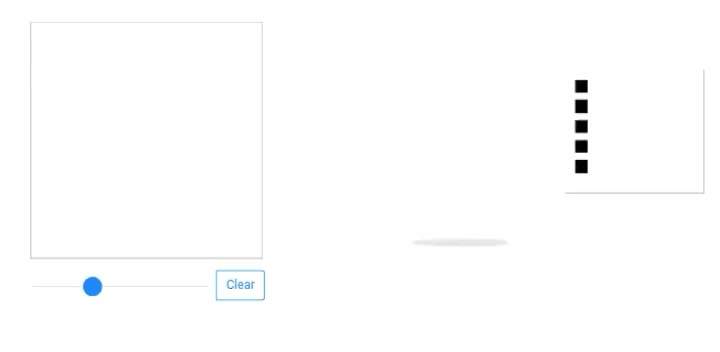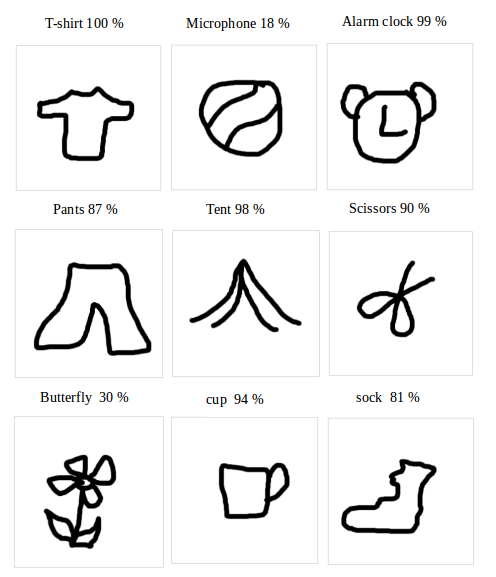Keras · TensorFlow.js ·Train a model in tf.keras with Colab, and run it in the browser with TensorFlow.js
July 02, 2018
Posted by Zaid Alyafeai

We will create a simple tool that recognizes drawings and outputs the names of the current drawing. This app will run directly on the browser without any installations. We will use Google Colab for training the model, and we will deploy it on the browser using TensorFlow.js.### Code and Demo

Find the live demo and the code on GitHub. Also make sure to test the notebook on Google Colab here.

### Dataset

We will use a CNN to recognize drawings of different types. The CNN will be trained on the Quick Draw dataset. The dataset contains around 50 million drawings of 345 classes.A subset of the classes

### Pipeline

We will train the model on GPU for free on Google Colab using Keras then run it on the browser directly using TensorFlow.js(tfjs) . I created a tutorial on TensorFlow.js. Make sure to read it before continuing. Here is the pipeline of the projectThe pipeline

### Train on Colab

Google provides free processing power on a GPU. You can see this tutorial on how to create a notebook and activate GPU programming.

#### Imports

we will use keras with tensorflow backend
``````import os
import glob
import numpy as np
from tensorflow.keras import layers
from tensorflow import keras
import tensorflow as tf``````

Since we have a limited memory we will not train on all the classes. We will only use 100 classes of the dataset. The data for each class is available on Google Cloud as numpy arrays of the shape `[N,784]` where `N` is the number of of the images for that particular class. We first download the dataset
``````import urllib.request

for c in classes:
cls_url = c.replace('_', '%20')
path = base+cls_url+'.npy'
print(path)
urllib.request.urlretrieve(path, 'data/'+c+'.npy')``````
Since our memory is limited we will only load to memory 5000 images per classes. We also reserve 20% of the data unseen for testing
``````def load_data(root, vfold_ratio=0.2, max_items_per_class= 5000 ):
all_files = glob.glob(os.path.join(root, '*.npy'))

#initialize variables
x = np.empty([0, 784])
y = np.empty()
class_names = []

#load a subset of the data to memory
for idx, file in enumerate(all_files):
data = data[0: max_items_per_class, :]
labels = np.full(data.shape, idx)

x = np.concatenate((x, data), axis=0)
y = np.append(y, labels)

class_name, ext = os.path.splitext(os.path.basename(file))
class_names.append(class_name)

data = None
labels = None

#separate into training and testing
permutation = np.random.permutation(y.shape)
x = x[permutation, :]
y = y[permutation]

vfold_size = int(x.shape/100*(vfold_ratio*100))

x_test = x[0:vfold_size, :]
y_test = y[0:vfold_size]

x_train = x[vfold_size:x.shape, :]
y_train = y[vfold_size:y.shape]
return x_train, y_train, x_test, y_test, class_names``````

#### Preprocess the Data

We preprocess the data to prepare it for training. The model will take batches of the shape `[N, 28, 28, 1]` and outputs probabilities of the shape `[N, 100]`
``````# Reshape and normalize
x_train = x_train.reshape(x_train.shape, image_size, image_size, 1).astype('float32')
x_test = x_test.reshape(x_test.shape, image_size, image_size, 1).astype('float32')

x_train /= 255.0
x_test /= 255.0

# Convert class vectors to class matrices
y_train = keras.utils.to_categorical(y_train, num_classes)
y_test = keras.utils.to_categorical(y_test, num_classes)``````

### Create the Model

We will create a simple CNN. Notice that the simpler the model with lesser number of parameters the better. Indeed, we will run the model after conversion on the browser and we want the model to run fast for prediction. The following model contains 3 conv layers and 2 dense layers.
``````# Define model
model = keras.Sequential()
input_shape=x_train.shape[1:], activation='relu'))
# Train model
model.compile(loss='categorical_crossentropy',
metrics=['top_k_categorical_accuracy'])
print(model.summary())``````

#### Fit, Validate and Test

After that we train the model for `5` epochs and `256 `batches with `10%` validation split
``````#fit the model
model.fit(x = x_train, y = y_train, validation_split=0.1, batch_size = 256, verbose=2, epochs=5)

#evaluate on unseen data
score = model.evaluate(x_test, y_test, verbose=0)
print('Test accuarcy: {:0.2f}%'.format(score * 100))``````And the testing accuracy is `92.20%` top `5` accuracy.

#### Prepare the model for Web Format

After we are satisfied about the accuracy of the model we save it in order to convert it
``model.save('keras.h5')``
we install the tfjs package for conversion
``!pip install tensorflowjs ``
then we convert the model
``````!mkdir model
!tensorflowjs_converter --input_format keras keras.h5 model/``````
This will create some weight files and the json file which contains the architecture of the model.

``!zip -r model.zip model ``
``````from google.colab import files

### Inference on the Browser

In this section we show how to load the model and make inference. I will assume that we have a canvas of size `300 x 300`. I will not go over the details of the interface and focus on TensorFlow.js part.

In order to use TensorFlow.js first use the following script
``<script src="https://cdn.jsdelivr.net/npm/@tensorflow/tfjs@latest"> </script>``
You will need a running server on your local machine to host the weight files. You can create an apache server or host the page on GitHub as I did on my project.

After that, load the model to the browser using
``model = await tf.loadLayersModel('model/model.json')``
The `await` keyword waits for the model to be loaded by the browser.

#### Preprocessing

We need to preprocess the data before making a prediction. First get the image data from the canvas
``````//the minimum boudning box around the current drawing
const mbb = getMinBox()
//cacluate the dpi of the current window
const dpi = window.devicePixelRatio
//extract the image data
const imgData = canvas.contextContainer.getImageData(mbb.min.x * dpi, mbb.min.y * dpi,
(mbb.max.x - mbb.min.x) * dpi, (mbb.max.y - mbb.min.y) * dpi);``````
The `getMinBox()` will be explained later. The variable `dpi` is used to stretch the crop of the canvas according to the density of the pixels of the screen.

We take the current image data of the canvas convert it to a tensor, resize and normalize
``````function preprocess(imgData)
{
return tf.tidy(()=>{
//convert the image data to a tensor
let tensor = tf.browser.fromPixels(imgData, numChannels= 1)
//resize to 28 x 28
const resized = tf.image.resizeBilinear(tensor, [28, 28]).toFloat()
// Normalize the image
const offset = tf.scalar(255.0);
const normalized = tf.scalar(1.0).sub(resized.div(offset));
//We add a dimension to get a batch shape
const batched = normalized.expandDims(0)
return batched
})
}``````
For prediction we use `model.predict` this will return probabilities of the shape `[N, 100]`
``const pred = model.predict(preprocess(imgData)).dataSync()``
We can then use simple functions to find the top 5 probabilities.

#### Improve Accuracy

Remember that that our model accepts tensors of the shape `[N, 28, 28,1]` . The drawing canvas we have is of size `300 x 300` which might be two large for drawings or the user might draw a small figure. It will be better to crop only the box that contains the current drawing. To do that we extract the minimum bounding box around the drawing by finding the top left and the bottom right points
``````//record the current drawing coordinates
function recordCoor(event)
{
//get current mouse coordinate
var pointer = canvas.getPointer(event.e);
var posX = pointer.x;
var posY = pointer.y;

//record the point if withing the canvas and the mouse is pressed
if(posX >=0 && posY >= 0 && mousePressed)
{
coords.push(pointer)
}
}

function getMinBox(){

var coorX = coords.map(function(p) {return p.x});
var coorY = coords.map(function(p) {return p.y});
//find top left corner
var min_coords = {
x : Math.min.apply(null, coorX),
y : Math.min.apply(null, coorY)
}
//find right bottom corner
var max_coords = {
x : Math.max.apply(null, coorX),
y : Math.max.apply(null, coorY)
}
return {
min : min_coords,
max : max_coords
}
}``````

### Testing Drawings

Here are some first time drawings and the highest percentage class. I made all the drawings with my mouse. You should get better accuracy using a pen …Next postPosted by Zaid Alyafeai

We will create a simple tool that recognizes drawings and outputs the names of the current drawing. This app will run directly on the browser without any installations. We will use Google Colab for training the model, and we will deploy it on the browser using TensorFlow.js.
Code and DemoFind the live demo and the code on GitHub. Also make sure to test the notebook on Googl…